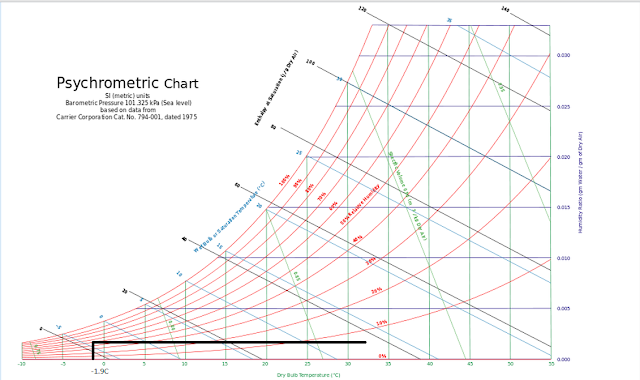## New Computer Fund## Sunday, June 17, 2012

### Defining the Moisture Boundary Layer

The Moisture Boundary Layer is the region where water exists only as a liquid or a vapor.  Adding salt or other impurities changes the freezing point of water.  This causes the MBL to have a variable limit that would need to be considered depending on the more dominate quantity, fresh or salt water.

As the temperature of the water increases, more water would evaporate.  The volume enclosed in the MBL would vary with the average temperature and energy contained in the MBL.  Since the volume of water that air can contain varies with temperature, an assumption that air inside the MBL on average contains 50% of its maximum capacity can be made.Minimum temperature and humidity of the moisture boundary layer
On the psychrometric chart above is the defined lower for the moisture boundary layer based on the freezing point of salt water and 50% relative humidity.  The vertical line from -1.9C (271.25K) stops at the 50% RH curve.  The horizontal line is the sensible heating/cooling process where there would be no change in the moisture content of the air, only the relative humidity.Moisture Boundary with average heat loop
Since we have an approximate Taverage, the average sea surface temperature of 294.25 K (21.1C) degrees and are using 50% RH as a baseline, the red line indicates the rise in moisture content for the high energy portion of the moisture cycle.  The blue line is the combined sensible and latent cooling from that maximum.  The low temperature end of the loop is not closed at this point since the chart does not consider the heat of fusion.  From the average energy point, the enthalpy of the air is approximately 41Joules per gram of dry air and the lowest point approximately 2 Joules per gram of dry air a difference of approximately 39J/g.

The sensible heat is equal to the specific heat capacity of the air time the change in temperature, for our atmosphere at sea level the specific heat of dry air is approximately 1 J/g at  sea level and the difference in temperature is 21.1-(-1.9)=23C.  The change in enthalpy, 39J/g is the total change in capacity resulting in a sensible heat ratio of 23/39=0.59 for the loop.

Using the Stefan-Boltzmann relationship, 294.25K has an energy of 425Wm-2, 271.25 has an energy of 306.9Wm-2.   There is a difference of 118.1Wm-2 which would be a steady state flow if the MBL was not changing or in equilibrium.  With a sensible heat ratio of 0.59, 0.59*118.1=69.6Wm-2 would be the sensible portion of the heat loss inside the MBL.  With the MBL radiating energy at a rate of 306.9 Wm-2 and it is receiving an average of 425Wm-2 from the interior, to remain in equilibrium it would have to radiate 118.1 Wm-2, the difference in the energy levels.  The internal energy flux of the MBL would be equal to the energy flux lost to the atmosphere beyond the MBL.  Assuming there is negligible water vapor beyond the MBL  118.1Wm-2 transferred through the MBL would be all sensible heat.  118.1-69.6=48.5Wm-2 may be purely radiant or a combination of conductive, convective and radiant.

Energy would also be transferred in the liquid water.  From 294.25K to 271.25K there is the 23C change in temperature, it requires 4.2J/g of energy to change water 1 degree C, that energy would be 4.2*23=96.6J/gram which would be 96.6Wm-2 per second by definition.

None of these rates of flows consider the total flow inside or outside the envelope of the Moisture Boundary layer.  They do provide some insight on the best way to proceed.

1. 96.6Wm-2 is a conductive flow rate within the water.  That would have a slower rate of flow than in the less dense atmosphere.

2. 118.1 Wm-2 is the approximate flow rate in the atmosphere from the average ocean surface temperature to the 271.25K lower temperature limit of the MBL.  This rate of flow is higher indicating that it would be more likely to determine the steady state conditions than the internal ocean flow rate.

3.  The psychometric chart estimate using the 50% relative humidity base indicates a Sensible Heat Ratio (SHR) of 0.59 and an approximate total enthalpy of 39J/g which can be used to estimate the to annual ice volume change for equilibrium conditions once total energy flows are estimated.

4. By defining the MBL lower limit as 271.25K and 50% relative humidity we can use conservation of energy for that thermal "envelop" to estimate the next thermal boundary layer which would have little to no latent heat, which should help simplify further calculations.

The next installment